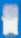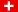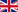DMX Address Setting - DMX Calculator

Many of our show laser light systems can be operated in DMX512 mode. As DMX is a serial signal with a special protocol, it is possible to apply a certain start address to every system in the line.

This calculation tool shall help you in finding the right dip-switch settings for a certain DMX address. The dip switches are set according to binary calculation meaning that every dip switch can feedback only the values 0 and 1. The calculation is comparably simple: Every dip switch has the value 2n where n is the number of the dip switch. First dip switch is 0 (computers start counting from 0, not from 1), so when it's switched on it says 20 = 1. Dip switch 2 has the value 1 if switched on, so it is 21 =2. Third dip switch has 22 = 4, fourth 23 = 8.
To Set a value you first have to find the highest number that fits the value, then you add up smaller values.

Example:
DMX512 value 11 shall be set:
1. Highest number that fits is 23 = 8
2. Second highest number is 21 = 2
3. third highest number that fits is 20 =1
So setting would be: 11010000

Input DMX Address Number:+41-71-6778080+44-161-8720272

[email protected]

laser light

laser light

Pangolin

Quickshow

Showeditor